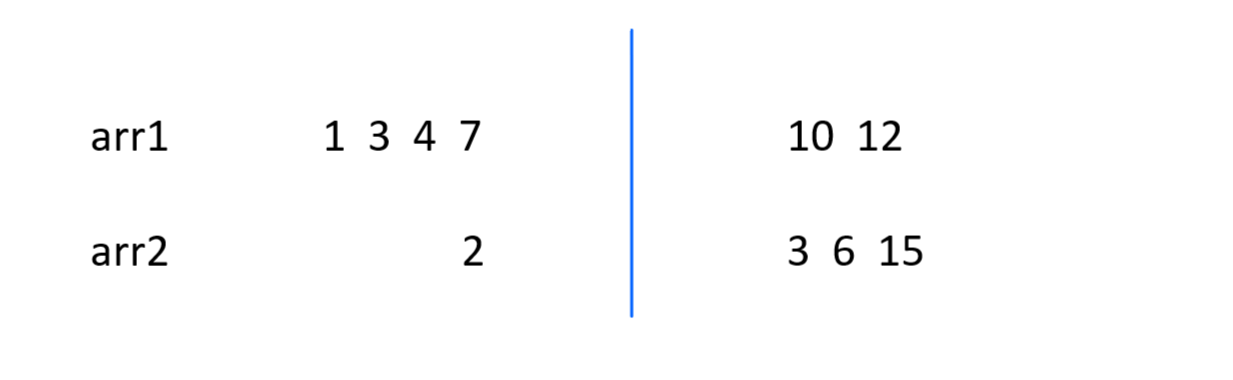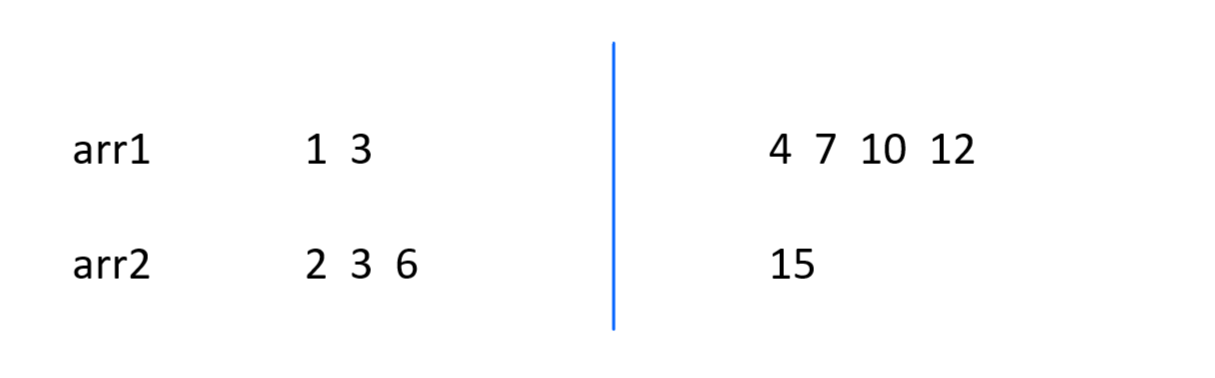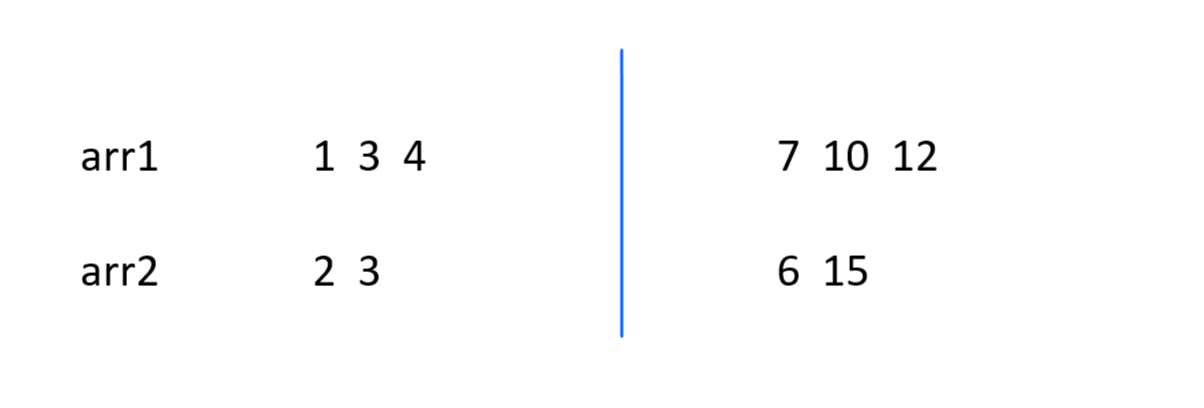# Median Of Two Sorted Arrays LeetCode Solution 2022Median Of Two Sorted Arrays LeetCode Solution: Hi there, fellow geeks! In this article, we will tackle a classic problem of binary search in which we are required to find the median of the array that results from merging two array into a sorted manner.

The problem statement says that we are given two arrays, A of size m and array B of size n, which are sorted in ascending order. The task is to merge the arrays and find the median of the merged array. Though it is not necessary to actually merge the arrays. Let us look at some methods to find the answer without consuming extra space.

Contents

### Explanation:

The problem statement says that we will be given arrays of unequal size as argument of the function that we need to complete. The arrays are sorted in ascending order. Our job is to find the median that is the median of the array that results if we somehow merge both the arrays. This median also depends on the number of elements in the merged array.

We will try to seek multiple solutions to this question without actually merging the arrays because that is a very brute and time consuming approach. The time complexity of such an algorithm will be at least O(m+n) where m is the length of the first array and n is the length of the second array. We will try to reduce the time complexity.

### Approach 1: (Using Linear Traversal)

We know that the arrays are sorted and the median will come from the middlemost element of the merged-sorted array. Thus it is easy to observe that we can traverse both the arrays using two pointers and store the elements that have been traversed into another array which will empty initially. This counting will stop when we have reached the central element of the merged array. Then, depending upon whether the final array contains even elements or odd elements, the median can be calculated easily. Let us put this method into an algorithm.

Algorithm:

1. Store the sum of lengths of both the arrays into n.
2. Declare an empty array merged of size n.
3. Initialize i = 0, j = 0, k = 0. These variables will serve as the index pointers.
4. Initialize a count variable to 0 and a current variable.
5. Repeat steps 6 to 8 while count is less than n.
6. If ith element of array1 is less than or equal to jth element of array2, store it in current and increment i.
7. Else if jth element of array2 is smaller than ith element of array1, store it in current and increment j.
8. Push back the value of current into the merged array and increment k.
9. If n is even, median is the average of the two middlemost element.
10. Else if n is odd, median is directly the central element.
11. Return the median value.

#### Implementation in C++:

```class Solution {
public:
double findMedianSortedArrays(vector<int>& nums1, vector<int>& nums2) {
int n = nums1.size() + nums2.size();
int merged[n];
int mid = n/2;
double median;
int i = 0, j = 0, k = 0;
int count = 0, curr;
while(count<n){
if(i<nums1.size() and j<nums2.size()){
if(nums1[i]<=nums2[j]){
curr = nums1[i++];
}
else{
curr = nums2[j++];
}
}
else if(i<nums1.size()){
curr = nums1[i++];
}
else{
curr = nums2[j++];
}
merged[k++] = curr;
count++;
}
if(n%2==0){
median = merged[mid] + merged[mid-1];
median /= 2;
}
else{
median = merged[mid];
}
return median;
}
};```

#### Analysis of the Algorithm:

This approach to the solution of Median Of Two Sorted Arrays is a brute force method. Time complexity for this code is O(m+n) where ‘m’ is the length if the first array and ‘n’ is the length of the second array. The space complexity for this code is O(m+n) because we require an additional array of size (m+n).

### Approach 2: (Using binary search)

Previous one was the easiest and most obvious approach. But this question lies in the difficult region and is meant to be solved using the binary search method. When talking about sorted arrays, the first algorithm that comes to mind is Binary Search. We know that the size of the merged array will be (m+n) where m is the size of the first array and n is the size of the second array. This approach may look like merge sort.

In the merged array if we make a partition along the central element or elements, the array will be divided into two halves. All the elements of the first half will definitely be smaller than all the elements of the second half. Using this property we will try to construct the the first and second halves. For this operation, we will select certain random elements from both the arrays.

As mentioned previously, all the elements on the right half subarray must be smaller than all the elements on the left half subarray. It can be observed that this condition is satisfied if that the last element of the right half is smaller than the first element of the left half.

To find an optimal median of two sorted arrays solution, we will select elements from both the arrays such that half the elements constitute the right half and the rest form the left half of the merged array. But obviously we cannot select random elements to form the required subarray. So we will need to check the condition given in the previous paragraph to find the correct sequence of elements.

Suppose,

arr1[] = {1, 3, 4, 7, 10, 12}

arr2[] = {2, 3, 6, 15}

The merged array for these two arrays will be,

arr[] = {1, 2, 3, 3, 4, 6, 7, 10, 12, 15}

Suppose, we select 4 elements from arr1 and 1 element from arr2 for the formation of the right half and 2 elements from arr1 and 3 elements from arr2 for the left half. Then the subarray must look like this for a random combination.To check whether all the elements of right half is less than all the elements of the left half, we must check and compare the extreme elements. Now, if we half selected the correct elements for the subarray, then 7 <= 3 and 2 <= 10. This condition is not true because 7 is not less than equal to 3. Thus, all elements of right half are not smaller than all elements of left half. This only means that the subarray that we selected are invalid. So, let us select some other combination. For example,In this case also the subarray are not valid because 6 is not less than or equal to 4. Thus these are not the halves we were looking for. Let us try another combination.It can be observed that in this combination of elements, 4<=6 and 3<=7. This means that all the elements on the right half are smaller than or equal to all the elements on the left half. Thus, this is the valid merged array that is the one we were looking for.

Thus, we checked the extreme elements of both the subarray to match the condition of a merged and sorted array. In the right half of the merged array, last element of arr1 is denoted by l1 and last element of arr2 is denoted by l2. In the left half of the merged array, first element of arr1 is denoted by r1 and first element of arr2 is denoted by r2. Therefore, the checking condition can be written as: ((l1 <= r2) and (l2 <=r1))

The mean of the merged array will be: (max(l1, l2) + min(r1, r2))/2

Now, to implement this approach to median of two sorted arrays using binary search, we just need to figure out where to make the partition in the arrays such that the checking condition is valid. We will find the partition using binary search by checking the condition for the extreme elements for each iteration. As per the binary search algorithm, the lower index will move downwards if the required element for partition should be smaller or it will move upwards if the required element for partition is larger.

#### Implementation in C++:

```class Solution {
public:
double findMedianSortedArrays(vector<int>& nums1, vector<int>& nums2) {
if(nums2.size() < nums1.size()) return findMedianSortedArrays(nums2, nums1);
int n1 = nums1.size();
int n2 = nums2.size();
int low = 0, high = n1;

while(low <= high) {
int cut1 = (low+high) >> 1;
int cut2 = (n1 + n2 + 1) / 2 - cut1;

int left1 = cut1 == 0 ? INT_MIN : nums1[cut1-1];
int left2 = cut2 == 0 ? INT_MIN : nums2[cut2-1];

int right1 = cut1 == n1 ? INT_MAX : nums1[cut1];
int right2 = cut2 == n2 ? INT_MAX : nums2[cut2];

if(left1 <= right2 && left2 <= right1) {
if( (n1 + n2) % 2 == 0 )
return (max(left1, left2) + min(right1, right2)) / 2.0;
else
return max(left1, left2);
}
else if(left1 > right2) {
high = cut1 - 1;
}
else {
low = cut1 + 1;
}
}
return 0.0;
}
};```

#### Implementation in JAVA:

```class Solution {
public double findMedianSortedArrays(int[] nums1, int[] nums2) {
if(nums2.length < nums1.length) return findMedianSortedArrays(nums2, nums1);
int n1 = nums1.length;
int n2 = nums2.length;
int low = 0, high = n1;

while(low <= high) {
int cut1 = (low+high) >> 1;
int cut2 = (n1 + n2 + 1) / 2 - cut1;

int left1 = cut1 == 0 ? Integer.MIN_VALUE : nums1[cut1-1];
int left2 = cut2 == 0 ? Integer.MIN_VALUE : nums2[cut2-1];

int right1 = cut1 == n1 ? Integer.MAX_VALUE : nums1[cut1];
int right2 = cut2 == n2 ? Integer.MAX_VALUE : nums2[cut2];

if(left1 <= right2 && left2 <= right1) {
if( (n1 + n2) % 2 == 0 )
return (Math.max(left1, left2) + Math.min(right1, right2)) / 2.0;
else
return Math.max(left1, left2);
}
else if(left1 > right2) {
high = cut1 - 1;
}
else {
low = cut1 + 1;
}
}
return 0.0;
}
}```

#### Analysis of the algorithm:

This solution to median of two sorted arrays uses binary search, so the time complexity for this code is O(log n) where ‘n’ is the total number of elements in the final merged array. This time complexity can also be O(1) for the best case that is, if we find the partition right away with the middle element. And the binary search space complexity is O(1) because it uses no additional data structure.

Also Read: How to Reverse an Array

### Conclusion:

This problem is an extension of median of arrays of equal size problem. It can be solved by a simpler approach if we directly merge the arrays while keeping the final array sorted. But the more efficient approach would be to use binary search because it uses no extra space and the time complexity is also significantly low.